Selection of Smoothing Parameters

### CV and GCV

The smoothers discussed here have a single smoothing parameter. In choosing the smoothing parameter, cross validation can be used. Cross validation works by leaving points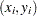out one at a time, estimating the squared residual for smooth function atbased on the remaining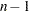data points, and choosing the smoother to minimize the sum of those squared residuals. This mimics the use of training and test samples for prediction. The cross validation function is defined aswhere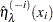indicates the fit at, computed by leaving out theth data point. The quantity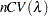is sometimes called the prediction sum of squares, or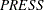(Allen 1974).

All of the smoothers fit by the GAM procedure can be formulated as a linear combination of the sample responses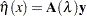for some matrix, which depends on. (The matrixdepends onand the sample data as well, but this dependence is suppressed in the preceding equation.) Let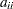be theth diagonal element of. Then the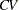function can be expressed as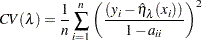In most cases, it is very time-consuming to compute the quantityindividually. To solve this computational problem, Wahba (1990) has proposed the generalized cross validation function (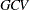) that can be used to solve a wide variety of problems involving selection of a parameter to minimize the prediction risk.

Thefunction is defined asTheformula simply replaces thewith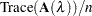. Therefore, it can be viewed as a weighted version of. In most of the cases of interest,is closely related tobut much easier to compute. Specify the METHOD=GCV option in the MODEL statement in order to use thefunction to choose the smoothing parameters.

### Degrees of Freedom

The estimated GAM model can be expressed as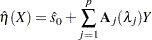Because the weights are calculated based on previous iteration during the local scoring iteration, the matrices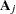might depend onfor non-Gaussian data. However, for the final iteration, thematrix for the spline smoothers has the same role as the projection matrix in linear regression; therefore, nonparametric degrees of freedom (DF) for theth spline smoother can be defined asFor loess smoothersis not symmetric and so is not a projection matrix. In this case PROC GAM usesThe GAM procedure gives you the option of specifying the degrees of freedom for each individual smoothing component. If you choose a particular value for the degrees of freedom, then during every local scoring iteration the procedure will search for a corresponding smoothing parameter lambda that yields the specified value or comes as close as possible. The final estimate for the smoother during this local scoring iteration will be based on this lambda. Note that for univariate spline and loess components, an additional degree of freedom is used by default to account for the linear portion of the model, so the value displayed in the “Fit Summary” and “Analysis of Deviance” tables will be one less than the value you specify.